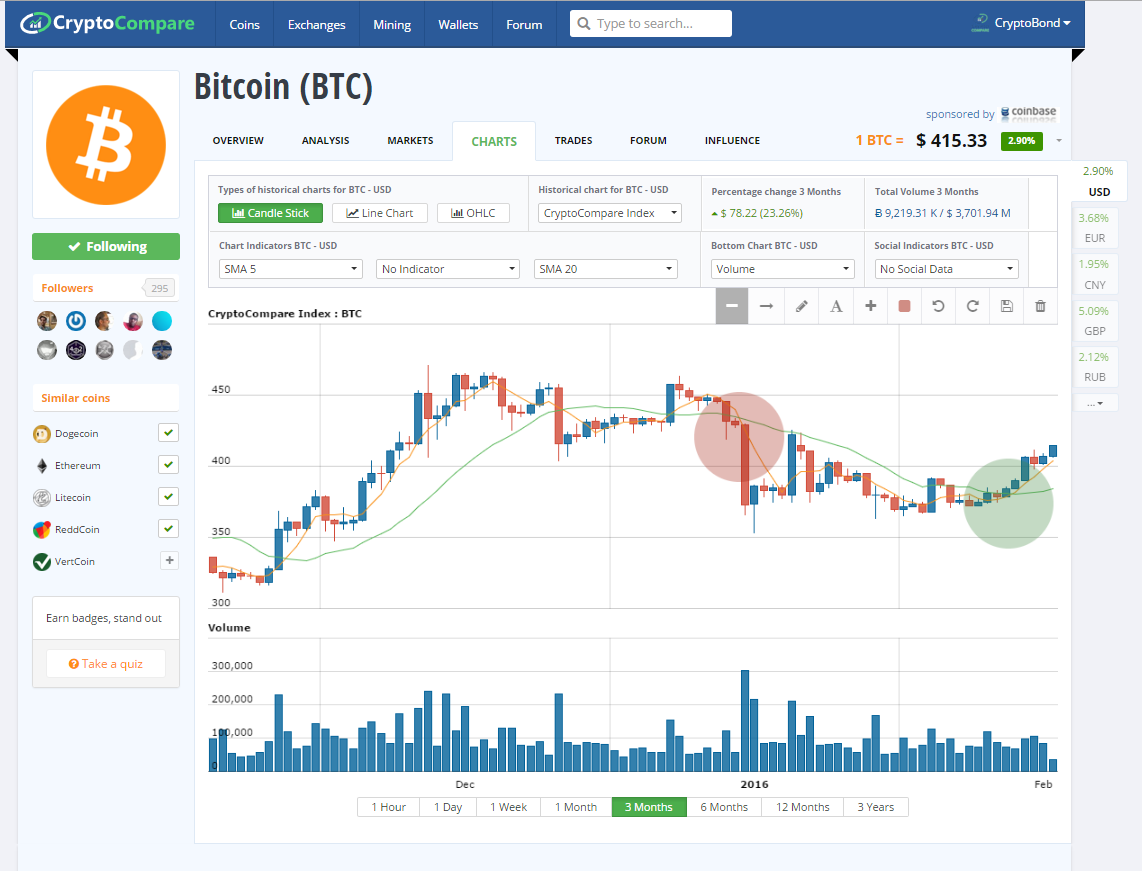# How to trade Bitcoin and other Crypto Currencies Using an SMA?

17 Feb 2016

The Simple Moving Average, SMA, is the most common indicator out there and is a definite must to understand for any aspiring technical analyst. Simple Moving  Averages, as their name suggests, are the generic moving average because of the way they are calculated.

What is an SMA?

An SMA is based on a particular time period so an SMA 14 is based on 14 periods whilst an SMA 30 is based on thirty periods. The larger the number of periods the less reactive the SMA is to recent price moves and as such the SMA is a momentum indicator as it moves based on price swings in the underlying.

How does the SMA Work?

The SMA is calculated by taking the number of periods required and summing the closing the price values at the close. So if you are calculating the SMA 12 for the price at 12 PM then take the price each hour before that until 1PM - then sum them up. The next step is to divide the total by the number of periods. It is just the average price for the number of periods.

Often simple moving averages are used in conjunction with each other to spot trend reversals and shifts in momentum. For example when a short term SMA is below a longer term one and then crosses it - you have indicated an upward shift in momentum that is a buy signal.

Example Chart SMA and AnalysisIf you look at the Bitcoin price chart above you can see two cross overs of SMA 5 (Yellow) and the SMA 20 (green). The first cross over shows the SMA 5 crossing over the SMA20 from above indicating a change in trend and an indication to sell. The second (green circle) is indicating a buy signal as the SMA 5 crosses the SMA 20 to the upside.